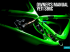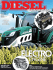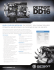# What Makes Boomerangs Come Back?

## Transcription

What Makes Boomerangs Come Back?
```What Makes Boomerangs Come Back?
Ernie Esser
UCLA
1
Parts of a Boomerang
2
Right-Handed vs Left-Handed
Right
Left
There is a difference between the two. They are in fact mirror images of each
other.
This presentation is geared towards right-handed boomerangs, which turn
counter-clockwise when thrown. Left-handed boomerangs, on the other hand,
turn clockwise.
3
Goals for the talk:
• Discuss the lift equation, why a boomerang generates lift and how these
lift forces produce torque on a boomerang
• Explain gyroscopic precession and why torque on a boomerang makes it
turn
• Estimate the characteristic radius of a boomerang’s flight path
• Explain a boomerang’s tendency to lay over as it flies
• Comment on how to construct a boomerang
• Discuss boomerang throwing and catching techniques
4
Aerodynamic Lift
An airfoil produces lift when the combined effects of its orientation (angle of
attack) and its shape cause oncoming air to be deflected downward.
Sketch of Streamlines
[Acheson, Elementary Fluid Dynamics]
Boomerang wing cross section
Bevel on lifting arm
5
The Lift Equation
Under some simplifying assumptions, the lift produced by a wing is
proportional to the area of the wing, the speed of the wing squared and the
density of air. We will use the following approximaton of lift force on a wing:
FLif t
1 2
= ρU CL A
2
ρ = density
U = speed
A = area
CL = lift coefficient
Remark: This model puts the complex dependencies that are hard to compute,
like the dependence of lift on boomerang shape, angle of attack, air viscosity,
etc., into a single constant CL .
Why is FLif t ∝ ρU 2 ?
6
Intuition from Oversimplification
Downwash from a helicopter
Recall Newton’s Second Law: force = rate of change of momentum
where momentum = mass · velocity
mass of air deflected per unit time ∝ ρU
change in deflected air’s vertical velocity ∝ U
⇒ FLif t ∝ ρU 2
7
A more local viewpoint
direction of decreasing pressure
Consider air following the top curve
of the airfoil in two dimensions
airflow
A downward force is required to accelerate the air this way.
mv 2
(think centripetal force r for a spinning mass on a string)
This force comes from a difference in pressure, which is decreasing in the
direction of the force.
Consequences:
• Expect lower than normal pressure near top of airfoil
• Expect tangential acceleration of oncoming air along top of airfoil
8
Bernoulli’s Equation
Pressure P and velocity u are related by Bernoulli’s equation
1
P + ρ|u|2 = c (for some constant c)
2
Assuming:
constant density ρ
zero viscosity µ (inviscid)
incompressible
irrotational (no vortices)
Comment: If the flow is rotational, the equation still holds but c can be
different on different streamlines.
To compute force on the airfoil, we can integrate P times the inward pointing
normal n along the boundary of the airfoil. Assuming u → αu when
U → αU , we again expect to have FLif t ∝ ρU 2 .
9
Another Viewpoint: Circulation
Under the previous assumptions, the solution to the equations for conservation
of momentum and mass in the domain surrounding the airfoil is not unique.
It is, however, determined by a quantity called circulation, Γ, that measures
the counterclockwise rotation of air about the airfoil.
Z
Γ=
u · ds
C
Γ is computed by adding up the tangential component of velocity u along the
boundary of the airfoil given by the curve C.
10
Viscous Effects Determine Circulation
For an airfoil with a corner at the trailing edge, all solutions to the inviscid
problem have a singularity in the velocity there except for one. Adding even
the tiniest amount of viscosity picks out the solution where a stagnation point
is at the corner. (Kutta Condition)
Zero Circulation
Negative Circulation
[Acheson, Elementary Fluid Dynamics]
• Equal transit time argument is false. Negative circulation implies air
moving even faster above airfoil.
• Need to include effect of viscosity to correctly model dynamics.
Conservation of angular momentum plus decreasing circulation requires
shedding of counterclockwise vortex.
11
Computing Lift and Drag
Assume uniform air speed U at infinity.
FDrag is the force on the airfoil in the direction of the oncoming air, and
FLif t is perpendicular to the flow.
FLif t = −ρU Γ
FDrag = 0
• Good approximation of lift on an airfoil when viscosity and angle of
attack are small
• Useless for computing drag because inviscid model doesn’t have any
tangential forces on airfoil
Note: To incorporate tangential shear forces due to viscosity,
F =
Z
C
(P − 2µD) · nds
where D is the deformation matrix and depends on rates of change of velocity
u.
12
Getting Back to Lift Equation
F Lift
U
L
Γ ∝ U L and
FLif t = −ρU Γ
⇒ FLif t ∝ ρU 2 L
So for a wing with uniform crossection we get back the lift equation:
FLif t
1 2
= ρU CL A
2
Note: If we measure or simulate FLif t we can compute CL .
13
Reynolds Number
General characteristics of the flow can often be determined from the unitless
Reynolds number Re defined by
Re =
Where
ρLU
.
µ
ρ = density
L = characteristic length scale
U = typical flow velocity
µ = dynamic viscosity
• Small Re ⇒ smooth, steady flow
• Large Re ⇒ turbulent, unsteady flow, and thin boundary layer
• Inviscid approximation can work when Re is large
Re for boomerang is between 104 and 105
Re for cruising jumbo jet wing is on the order of 107
14
2D Navier Stokes Simulation
The Navier Stokes equations for an incompressible fluid with constant density
ρ, constant viscosity µ, and no forcing terms are
ρ
∂u
+ ρ(u · ∇)u = −∇P + µ4u
∂t
∇·u=0
Simulate flow around 2D cross section of boomerang arm using
semi-Lagrangian finite element method.
Boundary Conditions:
Parabolic inflow on left side
Neumann boundary condition on right side
Zero velocity (no slip) on top, bottom and airfoil surface
Initial Condition:
Zero velocity
Note: Displayed results will be for a zoomed in region surrounding the airfoil
15
Triangularization of Domain
16
Simulation of |u| for max Uin = 10
Speed |u| from t = 0 to t = .04, FLif t and FDrag
Re = 27000 , speed and Fnet/100 plotted
0.04
0.03
0.02
0.01
0
−0.01
−0.02
−0.03
−0.04
0
0.02
0
2
0.04
0.06
0.08
0.1
0.12
maxUin = 10 , t = 0.04 , Flift = 1.8094 , Fdrag = 0.11486
4
6
8
10
12
14
0.14
16
18
0.16
20
17
Simulation of P for max Uin = 10
Pressure P from t = 0 to t = .04, FLif t and FDrag
Re = 27000 , pressure and Fnet/100 plotted
0.04
0.03
0.02
0.01
0
−0.01
−0.02
−0.03
−0.04
0
−100
0.02
−50
0.04
0.06
0.08
0.1
0.12
maxUin = 10 , t = 0.04 , Flift = 1.8094 , Fdrag = 0.11486
0
50
100
0.14
150
0.16
200
18
Simulation of |u| for max Uin = 14.14
Speed |u| from t = 0 to t = .04, FLif t and FDrag
Re = 38183.7662 , speed and Fnet/100 plotted
0.04
0.03
0.02
0.01
0
−0.01
−0.02
−0.03
−0.04
0
0.02
0
0.04
0.06
0.08
0.1
0.12
maxUin = 14.1421 , t = 0.04 , Flift = 2.8515 , Fdrag = 0.19318
5
10
15
20
0.14
0.16
25
19
Simulation of P for max Uin = 14.14
Pressure P from t = 0 to t = .04, FLif t and FDrag
Re = 38183.7662 , pressure and Fnet/100 plotted
0.04
0.03
0.02
0.01
0
−0.01
−0.02
−0.03
−0.04
0
0.02
−100
0.04
0.06
0.08
0.1
0.12
maxUin = 14.1421 , t = 0.04 , Flift = 2.8515 , Fdrag = 0.19318
−50
0
50
100
150
0.14
0.16
200
20
Simulation of |u| for max Uin = 5
Speed |u| from t = 0 to t = .04, FLif t and FDrag
Re = 13500 , speed and Fnet/10 plotted
0.04
0.03
0.02
0.01
0
−0.01
−0.02
−0.03
−0.04
0
0.02
0
1
0.04
0.06
0.08
0.1
0.12
maxUin = 5 , t = 0.04 , Flift = 0.14187 , Fdrag = 0.025632
2
3
4
5
6
7
0.14
8
9
0.16
10
21
Simulation of P for max Uin = 5
Pressure P from t = 0 to t = .04, FLif t and FDrag
Re = 13500 , pressure and Fnet/10 plotted
0.04
0.03
0.02
0.01
0
−0.01
−0.02
−0.03
−0.04
0
0.02
−20
0.04
0.06
0.08
0.1
0.12
maxUin = 5 , t = 0.04 , Flift = 0.14187 , Fdrag = 0.025632
−10
0
10
20
30
0.14
40
0.16
50
22
• Can see starting vortex shed off trailing edge
• Pressure clearly lower above airfoil
• Velocity also larger near top surface of airfoil
• Thin boundary layer visible
• Earlier boundary layer separation for Re = 13500 suggests inviscid
approximation not as good for Re in that range or smaller
• Difficult to make simulation stable and accurate for high Reynolds
number flows
• Simulated FLif t not exactly following the FLif t ∝ U 2 model, but we’ll
call it close enough
23
A Free-Body Diagram
If we assume the drag force is negligible, then the only forces we consider are
the lift force and the force due to gravity.
Assume the boomerang travels in a circular path and let the vertical
component of the lift force balance gravity. Then we are left with a net force
that is parallel to the ground and can be thought of as a centripetal force.
24
A Top View of Boomerang Flight
Ω = rate of precession in
Fnet = centripetal force
R
boomerang’s flight path
So why does the boomerang turn?
25
Some Rotational Dynamics
The torque about a point c is defined by
τ =r×F
where F is the force and r is the vector from c to the point where the force is
applied. Torque is a vector and can be thought of in terms of its magnitude
and a direction. It’s magnitude is defined by
|τ | = |r||F| sin θ
where θ is the angle between the vectors r and F.
26
Right Hand Rule
The direction of the torque is perpendicular to both r and F. This direction is
determined by the right hand rule:
Point the fingers of the right hand in the direction of r and curl them in the
direction of F. Then the thumb will point in the direction of the torque τ .
F
r
c
torque points into page
[Bedford and Fowler, Engineering Mechanics]
27
Moment of Inertia
We also have the moment of inertia, I, which describes how difficult it is to
rotate a body about some axis. A boomerang, which rotates about it’s center
of mass, will retain its spin better if it has a high moment of inertia.
m
r
A particle of mass m rotating about an axis
at a distance of r, has moment of inertia
I = mr 2 .
A cylinder of mass m and radius r spinning
about its axis of symmetry has the following moment of inertia:
I=
1 2
mr
2
28
Angular Velocity
The angular velocity, ω, describes the rotation of a body about an axis. ω
points in the direction of the spin axis and its magnitude is the rate of rotation
For a particle, the relationship between the angular velocity and the linear
velocity is given by
v =ω×r.
v
r
w
w points out of screen
29
Angular Momentum
The angular momentum, J, is the rotational analogue to linear momentum.
Angular momentum is conserved in the absence of external torque, analogous
to how momentum is conserved in the absence of external forces. For a
particle, angular momentum is given by
J=r×p
where p is the linear momentum of the particle and r is it’s location with
respect to some choice of origin.
For an object that is symmetrical
about its axis of rotation, the following formula for angular momenJ = Iω
tum applies:
z
J
r
x
This formula also applies to planar
bodies, such as a boomerang, rotating about a perpendicular axis. We
p points into screen take the center of mass to be the origin and let I refer to the moment of
y
through the center of mass.
30
Principal Axes
For any rigid body, we can always find three perpendicular axes such that
when the object is spinning about one of those axis, its angular momentum is
in the same direction as its angular velocity.
(This is the spectral theorem applied to the symmetric inertia matrix.)
• For a boomerang in the x-y plane, the z-axis is a principle axis.
• Its moment of inertia Iz about that axis is larger than about any other axis
y
x
m
Iz = m(x2 + y 2 ) = Iy + Ix
y
x
31
Relating torque and angular momentum
τ=
dJ
dt
In words, torque is the rate of change of the angular momentum. This
relationship between τ and J follows from Newton’s second Law, f =
which says force is the rate of change of linear momentum.
dp
dt ,
To simplify, consider a system of masses mi in a reference frame whose
origin coincides with the center of mass and which is not moving relative to
the center of mass.
J
dJ
dt
=
=
=
=
P
i ri × pi
P
i vi × pi + ri × fi
P
i ri × f i
τ
32
Gyroscopic Precession
The equation τ = dJ
dt says that when a torque is applied to a rotating object
such as a boomerang, the angular momentum vector will change so that it
points more in the direction of the torque.
DEMO
The rate of precession Ω is related to torque and angular momentum by
J
boomerang
τ =Ω×J.
points out of screen
33
Why is there torque on a boomerang?
The boomerang is moving with linear velocity V and spinning with angular
velocity w.
This means the top of the boomerang is moving faster than the bottom. Since
lift is proportional to the velocity squared, the top part generates more lift than
the bottom part. This results in a net torque!
34
Which way does the boomerang turn?
If we draw the boomerang in the x-y plane traveling in the negative
x-direction with the angular momentum vector pointing in the z-direction, we
see that the torque points in the positive x-direction.
Apply the equation τ =
dJ
dt .
35
It can be shown a that the magnitude of torque on a boomerang is roughly
proportional to the product of its linear and angular velocities.
τ ∝ vω
Recalling the relationship between torque and the rate of precession, we have
|τ | = |Ω × Iω| = cvω
for some constant c.
Canceling ω implies Ω = c̃v for some constant c̃. And since we also know
v
, where R is the radius of the boomerang’s flight, we see we can solve
Ω= R
for R and that is it independent of ω and v.
R=
1
c̃
Note: If we can compute τ we can compute R.
a
[Felix Hess, The Aerodynamics of Boomerangs]
36
Compute torque for Roomerang
The Plan:
Use the lift equation to compute the lift
force and torque produced by a fourbladed windmill-shaped boomerang. Then
estimate the characteristic radius of the
boomerang.
• Symmetrical windmill-shaped
boomerang
• Constant layover angle φ
Assumptions:
D
a
• Boomerang travels in level circle
• Lift equation FLif t = 1 ρU 2 CL A
2
applies
• Lift coefficient CL constant along
wings of boomerang
37
Making Use of Symmetry
Here we are concerned with the torque about the x-axis, which is the torque
that causes the main precession of the boomerang.
We will simultaneously consider the contribution to torque about the x-axis
from each of the 4 arms.
y
rw + vcos
w
v
rsin
x
rcos
a
38
Let j = 1, 2, 3, 4 index the four arms of the boomerang.
P
j τj (r, θ)dA =
=
=
P
1
2
ρC
v
(r,
θ)
yj dA
L
j
j 2
1
2 ρCL dA[r cos θ(v cos θ
2
+ rω)2 + r sin θ(v sin θ + rω)2
−r sin θ(v sin θ − rω) − r cos θ(v cos θ − rω)2 ]
2ρCL r 2 ωvdA
By symmetry, τ does not depend on θ.
Now integrating in polar coordinates gives the total torque
τ
Ra
= 2DρCL ωv 0 r 3 dr
4
= D
ρC
a
ωv
L
2
Thus τ is proportional to ωv.
39
Solving for R
Recall that Ω =
v
R
and τ = Ω × J.
Since J = Iω and the layover angle is assumed to be φ, we get
τ
Ω=
.
Iω cos φ
Next plug in our formula for torque,
v
DρCL a4 ωv
=
R
2Iω cos(φ)
⇒
D
τ = ρCL a4 ωv .
2
R=
2I cos φ
DρCL a4
We can get a more specific estimate for the windmill boomerang by
substituting I =
1
2
ma
,
2
D=
π
8
and cos φ =
√
3
2 .
Then
√
4 3m
.
R=
πa2 ρCL
40
Implications of the equation for R
R=
2I cos φ
DρCL a4
• Under our assumptions, R is independent of v and w. Thus the range of
a boomerang doesn’t depend on how it is thrown. R is instead a property
of the boomerang itself.
• A larger moment of inertia implies a bigger radius.
• Lower air density results in a larger radius.
• More wing area gives the boomerang a smaller flight radius.
• A larger lift coefficient also causes reduced range.
41
An Interesting Inverse Problem
The lift coefficient is extremely difficult to explicitly compute. Fortunately,
our equation for R gives us an easy way to determine CL experimentally.
CL =
1. Solve equation for CL .
kg
ρ ≈ 1.2 m
3
2. Measure air density.
3. Find mass of Roomerang.
4. Estimate wing angle D and wing radius a.
5. Throw boomerang and measure R.
6. Plug everything in.
√
4 3m
πa2 ρR
D≈
π
8
m ≈ .005kg
and a ≈ .14m
R ≈ 1m
CL ≈ .45
This gives us a rough approximation for the lift coefficient of the Roomerang.
42
Extension to Classical Boomerang Shape
Without special symmetry, the lift force and torque produced by the
boomerang depend on the angle of rotation. Felix Hess’s lift diagram below
illustrates the non-symmetric lift force for the lifting arm as it sweeps out a
circle. (The darker color corresponds to more lift.)
[Felix Hess, The Aerodynamics of Boomerangs]
The situation can be simplified by using time averages of lift and torque to
approximate the previous calculations.
43
What Makes a Boomerang Lay Over?
y
w
rw + vcos
x
v
rcos
rsin
a
A torque about the y-axis causes the boomerang to precess about the x-axis.
Thus a positive torque in the y-direction will cause the boomerang to lay over.
44
The Drafting Effect
The leading arms of boomerangs disturb the air so that the trailing arms
generate less lift than they normally would. There is a resulting torque in the
positive y-direction. The drafting effect is the most significant source of this
torque because it affects even radially symmetric boomerangs.
45
Without this drafting effect, our Roomerang generates no torque in the
y-direction. Analogous to our earlier computation
P
j τj (r, θ)dA =
=
=
P
− j 21 ρCL vj (r, θ)2 xj dA
1
2
2
2 ρCL dA[r sin θ(v cos θ + rω) −r cos θ(v sin θ + rω)
+r cos θ(v sin θ − rω)2 −r sin θ(v cos θ − rω)2 ]
0.
With less lift on the trailing arms, the two red terms actually have less effect.
This results in a positive torque in the y-direction.
46
Eccentricity Torque
Eccentricity torque is only an issue with non-radially symmetric boomerangs,
and is caused when there is unequal lift on arms with nonzero eccentricity.
The eccentricity refers to the perpendicular distance between an arm and the
center of mass.
If the lifting arm generates more lift than the dingle arm, the resulting torque
in the y-direction causes the boomerang to lay over faster.
47
Boomerang Construction and Tuning
Tools and Supplies:
Warp-resistant plywood, coping saw (or band
saw!), drill sander, wood file, knife, sandpaper,
and patience.
Woodworking
Irreversibility:
Err on the side of removing less wood for the
first test flight, because it’s easy to take wood
off but challenging to put it back on.
Distribution:
• Adding mass to the ends of arms
increases the moment of inertia resulting
in longer range and better wind
resistance.
• Adding mass to center improves wind
resistance and stability.
• A lot of very fine tuning can be accomplished by adding small weights to various parts of a boomerang.
48
• We can alter the shape of the bevels and airfoils to increase or decrease
the lift generated.
• More lift on the lifting arm yields a faster layover.
• More lift on the dingle arm results in less layover and a flatter, more
circular flight.
• Larger bevels increase lift and torque, causing the boomerang to make a
tighter turn.
• Too much lift can cause too tight of an orbit.
• Streamlining is important and can sometimes save a boomerang that
prematurely runs out of spin.
• Conversely, a rougher surface isn’t necessarily bad and can occasionally
improve flight characteristics.
49
Warping Effects
A warped boomerang is not necessarily a broken boomerang.
• A warped boomerang that is concave up will have reduced range, but a
concave down boomerang might not fly at all.
• The arms of a boomerang can be twisted to give them a positive or
negative angle of attack.
• More fine tuning can also be accomplished by turning the tips of the
arms up or down.
• A warping caution: I’ve found that intentionally warping a boomerang is
often only a temporary change, and the results can be inconsistent.
50
General Throwing Tips
Two Throwing Grips:
[Aboriginal Steve’s boomerang
page]
The Throwing Angle
51
Throwing and Troubleshooting
• The contoured side of the boomerang needs to be facing you.
• Throw boomerang directly ahead or slightly upward with moderate
power and spin.
• Use a layover angle of about 30 degrees or less. Do not throw sidearm.
• In windy conditions, throw about 45 degrees to the right of the oncoming
wind (to the left for left-handers using a left-handed boomerang).
Troubleshooting the Throw
Lands too far in front
Aim lower or throw harder
Lands too far behind
Aim higher or throw softer
Lands to the left
If wind, aim more to the right
Lands to the right
If wind, aim more into it
Flies too high and lands in front Use less layover
Hits ground too soon
Aim higher or throw harder
Just doesn’t quite make it back
Try more layover, power and/or spin
52
A Word on Catching and Safety
A Good Catch
Throw in a large open area devoid of potential boomerang victims (people,
windows, anything expensive) as well as boomerang predators (power lines,
trees, pit bulls). Only one boomerang at a time should be in the air so that no
one’s attention is dangerously divided.
53
DO NOT GET DISTRACTED!
Always keep your eyes on a thrown boomerang. It has a tendency to come
back. Either catch it, dodge it or protect yourself!
54
References
• Acheson, D.J. Elementary Fluid Dynamics, 2005.
• Boomerang Passion [http://www.boomerangpassion.com/english.php]
[http://www.flight-toys.com/boomerang/europe.htm]
• Hawes, Lorin Lindley and Mauro, John, All About Boomerangs, 1987.
• Hess, Felix, The Aerodynamics of Boomerangs, 1968.
• Hunt, Hugh, Unspinning the Boomerang
• Landau and Lifshitz, Mechanics
• Majda, Andrew J. and Bertozzi, Andrea L. Vorticity and Incompressible
Flow, 2002.
• Pakalnis, Saulius, Aerodynamics of Boomerang,
[http://researchsupporttechnologies.com/boomerang_site/boomerang1.htm]
• Persson, Per-Olof, and Strang, Gilbert.
• Reid, Robert, The Physics of Boomerangs, 1984.
55
```

### The Boomerang from Obtazowa and its Prehistoric Context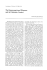### USA ELAN Manual - Boomerang RC Jets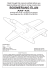### - Flight Toys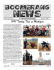### The Boomerang - EAA Chapter 40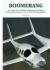### ETP Techno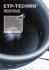### Boomerang REST API Specification.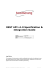### STEM and Project-Based Learning: What`s All the Excitement About?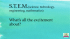### British Car Fayre Scheduled for September 8th in Norcross Summer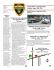### View BendPak vs Backyard Buddy Lift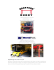### sb6c owner`s manual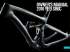### owner`s manual yeti sb6c